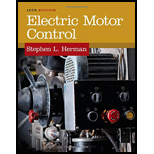# In Figure 40–2, approximately what is the percentage of current drawn from the line when the field current is adjusted to 100 percent of its capacity at full motor load?### Electric Motor Control

10th Edition
Herman
Publisher: CENGAGE L
ISBN: 9781133702818

#### Solutions

Chapter
Section### Electric Motor Control

10th Edition
Herman
Publisher: CENGAGE L
ISBN: 9781133702818
Chapter 40, Problem 7SQ
Textbook Problem
1 views

## In Figure 40–2, approximately what is the percentage of current drawn from the line when the field current is adjusted to 100 percent of its capacity at full motor load?

To determine

What percentage of current is drawn by the motor from the line in full load condition in Figure 40-2.

### Explanation of Solution

Refer to Figure 40.2 in the textbook; the graph is plotted between percentage of load line current and percentage of rated field current for a motor. In graph, if the motor field current is adjusted to 1...

### Still sussing out bartleby?

Check out a sample textbook solution.

See a sample solution

#### The Solution to Your Study Problems

Bartleby provides explanations to thousands of textbook problems written by our experts, many with advanced degrees!

Get Started

Find more solutions based on key concepts
List the steps that should be followed to precisely tighten a bolt to specifications.

Automotive Technology: A Systems Approach (MindTap Course List)

Name at least two units that you use every day.

Engineering Fundamentals: An Introduction to Engineering (MindTap Course List)

CAPS LOCK and NUM LOCK are two examples of toggle keys.

Enhanced Discovering Computers 2017 (Shelly Cashman Series) (MindTap Course List)

If your motherboard supports ECC DDR3 memory, can you substitute non-ECC DDR3 memory?

A+ Guide to Hardware (Standalone Book) (MindTap Course List)